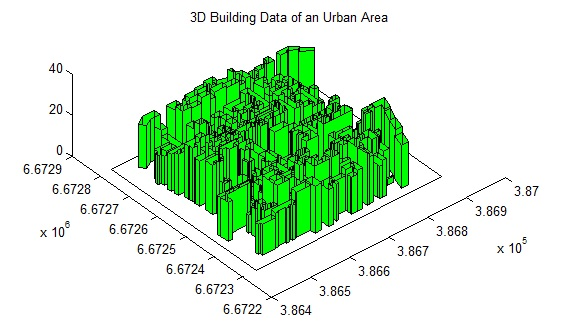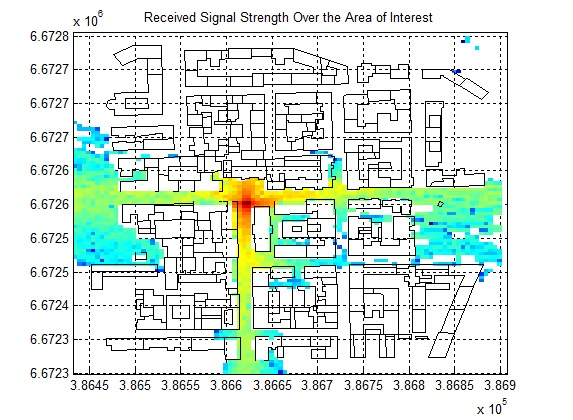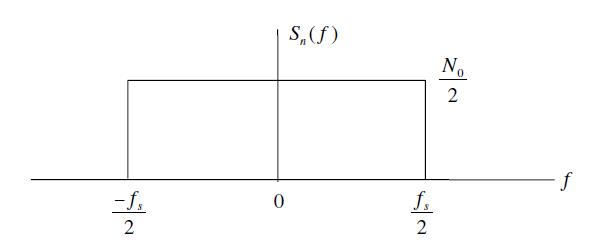# Pulse Amplitude Modulation Symbol Error Rate in AWGN

Pulse Amplitude Modulation (PAM) is a one dimensional or in other words real modulation. Simply put it is an extension of BPSK with M amplitude levels instead of two. This can be a bit confusing because BPSK can be looked at as a phase modulation and its natural extension must be QPSK or 8-PSK modulations. To remove this ambiguity lets call M-PAM an extension of simple amplitude modulation but with M levels. In the discussion below we consider M=4 but then extend it to the general case of M=2k (k=1,2,3…).

Continue reading Pulse Amplitude Modulation Symbol Error Rate in AWGN

# Eclipse

#### Eclipse 1.0 – A Paradigm Shift in RF Planning

NEW: Simulation of a Moving Transmitter (such as a car)

NEW: Simulation of a Moving Transmitter (such as a pedestrian)

Radio frequency planning is an essential component of network planning, roll-out, up-gradation, expansion etc. Several methods can be adopted for this from something as simple as free space models, empirical path loss models to the significantly more complicated, time consuming and expensive drive testing. Drive testing gives very accurate results but these results can be rendered useless by changing the position of an antenna or the tilt or transmit power of an antenna requiring another run in the field. One solution to this problem is ray-tracing which is very accurate but is usually considered to be very computationally expensive and of little practical value. But recent advances in computational power of machines coupled with efficient techniques have given a new lease of life to this method.

Eclipse is a near real-time simulation software for prediction of signal strength in urban areas. The software uses shooting and bouncing ray (SBR) method of ray tracing with 1 degree ray separation, 1 m step size and 9 interactions per ray path. The simulation parameters can be varied according to the resolution required. The code is highly optimized to give results in shortest possible time. It is especially useful for network planning of ultra-dense wireless networks where a dense network of antennas is placed on lamp posts instead of telecom towers. Various frequency bands can be simulated, along with different antenna radiation patterns and MIMO configurations.Note: If you would like to run a test simulation send us a request at info@raymaps.com

# Noise Calibration in Simulation of Communication Systems

We have been using a wireless signal model in our simulations without going into the details of noise calibration for simulation. In this article we discuss this. Lets assume the received signal is given as

r(t)=s(t)+n(t)

where r(t) is the received signal s(t) is the transmitted signal and n(t) is the Additive White Gaussian Noise (AWGN). Channel fading is ignored at the moment. Signal to noise ratio for simulation of digital communication systems is given as

ρ=Eb/No (1)

Where Eb is the energy per bit and No is the noise Power Spectral Density (PSD). We also know that for the case of Additive White Gaussian Noise the noise power is given as [Tranter]

σ^2=(No/2)*fs

No=2*(σ^2)/fsWhere σ is the standard deviation of noise and fs is the sampling frequency.  Substituting in equation 1 we get

ρ=Eb/No=Eb/(2*σ^2/fs )

Eb/No=(Eb*fs)/(2*σ^2)

σ^2=(Eb*fs)/(2*Eb/No )

σ=√((Eb*fs)/(2*Eb/No ))

If the energy per bit and the sampling frequency is set to 1 the above equation reduces to

σ=√(1/(2*Eb/No ))

The simulation software can thus calculate the noise standard deviation (or variance) for each value of Eb/No in the simulation cycle. The following piece of MATLAB code generates AWGN with the required power and adds it to the transmitted signal.

```s=sign(rand-0.5);      % Generate a symbol
sigma=1/sqrt(2*EbNo);  % Calculate noise standard deviation
n=sigma*randn;         % Generate AWGN with the required std dev
r=s+n;                 % Add noise to the signal```

How can we assume that energy per bit and sampling frequency is equal to one and are we breaking some discrete time signal processing rule here. This will be discussed in a later post.

# Ray-Tracing for Network Planning-II

It’s very easy to get lost in the jargon when selecting a simulation tool for planning your wireless network. You will be faced with complex terminology which would not make much sense. At one end of the spectrum are solutions based on simple empirical models while at the other end are solutions based on ray-tracing techniques. Empirical models are based on measurement data and are your best bet if you want a quick and cheap solution whereas ray-tracing techniques are based on laws of physics and promise more accurate results. In principle ray-tracing techniques are quite simple: just transmit a bunch of rays in all directions and see how they behave. However when the number of rays and their interactions becomes large the simulation time may become prohibitively expensive. The simulation time for complex geometries may vary from a few hours to several days.

Following are some of the factors that you must consider when selecting a ray-tracing simulator.

1. Upper limit on the number of interactions

Ray-tracing simulators essentially generate a bunch of rays (image based techniques are an exception) and then follow them around as they reflect, refract, diffract and scatter. Each interaction decreases the strength of the rays. The strength of the rays also decays with distance. As a result the simulator needs to decide when to terminate a ray path. This is usually done based upon the number of interactions that a ray undergoes (typically 8-10 interactions are considered) or based upon its strength (once the strength of a ray falls below -110 dBm there is no point following it any further). Higher the number of interactions considered, greater the accuracy of the simulation but higher the computational complexity.

2. Granularity in field calculations

Field calculations cannot be performed at each and every point within the simulation space. The usual approach is to divide the region under study into a grid such that locations closer to a transmitter are covered more finely and the regions further away are covered in lesser detail. The rays are then combined within each block of the grid to get the resultant field strength. The level of granularity determines the computation load. It would be prohibitively expensive to have a very high level of granularity for a large network.

3. Accuracy in modeling the various propagation phenomenon

As mentioned previously an accurate modeling of all propagation phenomena is required including reflection, refraction, diffraction and scattering. Some ray-tracing simulators might model reflection and refraction only while ignoring the other phenomenon such as diffraction. Furthermore some ray-tracing simulators might consider all reflections to be specular (no scattering). This is a good approximation for large smooth surfaces but is not such a good assumption for irregular terrain.4. Granularity of the terrain database

Most state of the art ray-tracing tools use some sort of terrain database to perform their calculations. These terrain databases are required for determining the paths of the rays as they travel in dense urban environments. These databases may contain simple elevation data or actual 3D building data. These databases may have accuracy of 10m or 30m or maybe more. The accuracy of the simulation is highly dependent on the granularity of the terrain database.

5. Accuracy in representation of building materials

The wireless signal propagation within cities is governed by complex phenomena such as reflection, refraction, diffraction and scattering. Let’s take the example of the phenomenon of reflection. The percentage of signal reflected back at a particular interface is dependent on permittivity and permeability of the object. Based on these properties only 10% of the signal maybe reflected or 50% of the signal may be reflected. So, for accurate simulation not only should we have a high level of granularity of the 3D building data, we also need an accurate description of the building materials.

6. Dynamic Channel Behavior

A wireless channel is continuously changing i.e. the channel is dynamic (as opposed to being static). However the ray-tracing techniques available in the literature do not capture this dynamic behavior. The dynamic behavior of the channel is mainly due to the motion of the transmitter or receiver as well as motion of the surroundings. While the position of the transmitter and receiver can be varied in the ray-tracing simulation the surroundings are always stationary. Hence a ray-tracing simulator is unable to capture the time-varying behavior of the channel.

The accuracy of ray-tracing simulators is bound to increase as the computational power of computers increases and as accurate 3D building databases become available throughout the world. Until that time we would have to fall back to approximate simulations or maybe measurement results.

# Ray-Tracing for Network Planning-I

It’s very easy to get lost in the jargon when selecting a simulation tool for planning your wireless network. You will be faced with complex terminology which would not make much sense. You will be told that ray-tracing is the solution to all problems and outperforms all other techniques. However ray-tracing is only accurate when the following factors have been considered.

1. Granularity of the terrain database
2. Granularity in field calculations
3. Accuracy in representation of building materials
4. Accuracy in modeling the various propagation phenomenon
5. Upper limit on the number of interactions